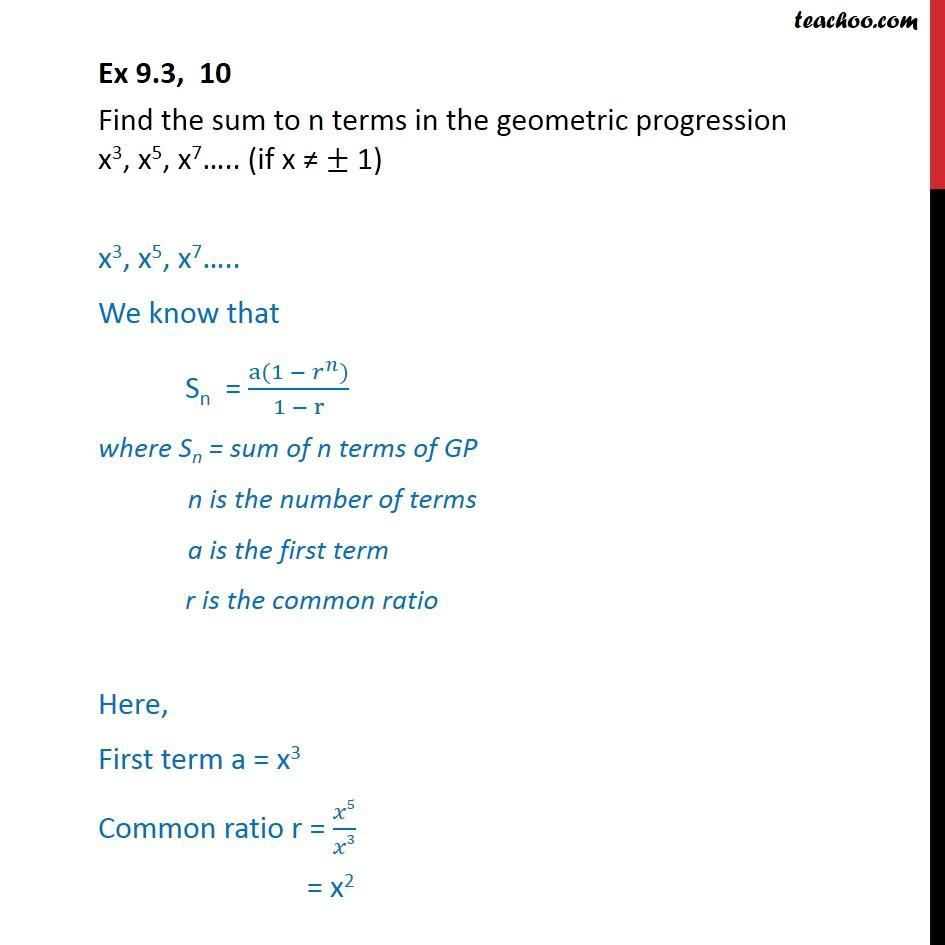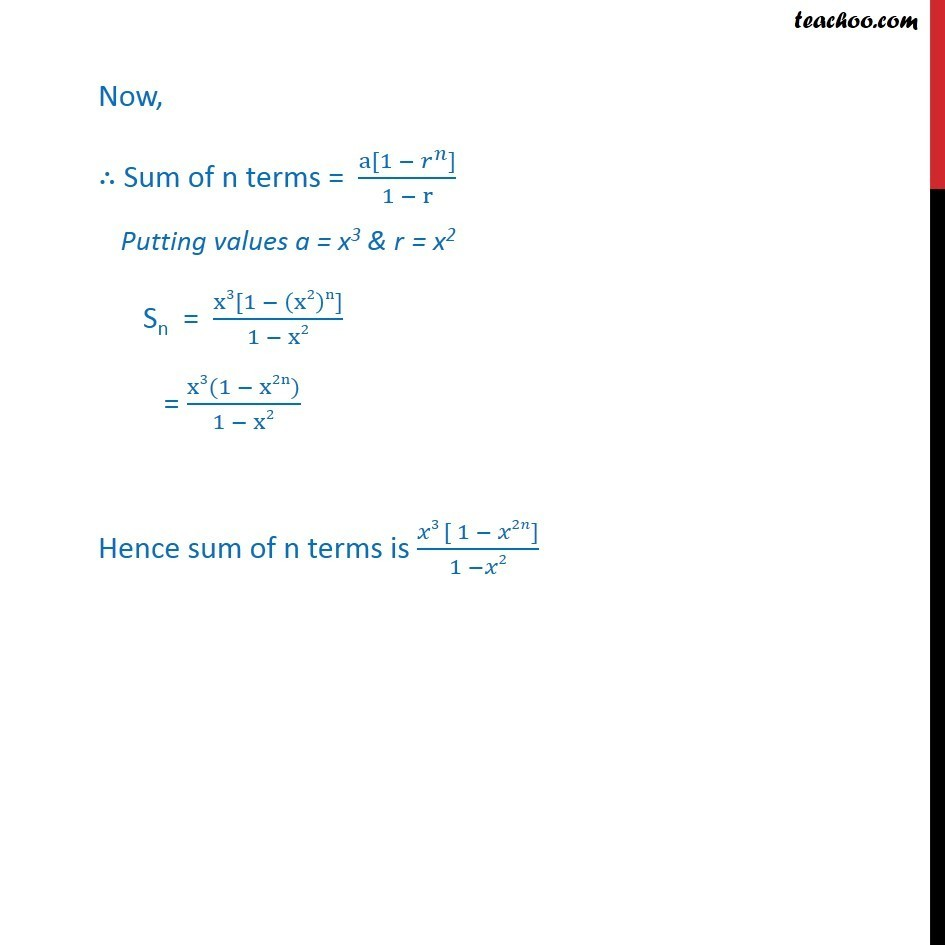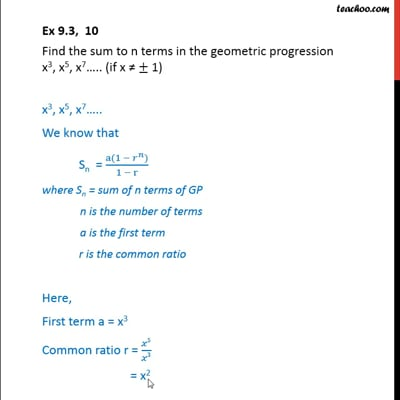Ex 9.3

Chapter 9 Class 11 Sequences and Series
Serial order wiseThis video is only available for Teachoo black users

Solve all your doubts with Teachoo Black (new monthly pack available now!)

### Transcript

Ex 9.3, 10 Find the sum to n terms in the geometric progression x3, x5, x7….. (if x ≠ ± 1) x3, x5, x7….. We know that Sn = (a(1 − 𝑟^𝑛))/(1 − r) where Sn = sum of n terms of GP n is the number of terms a is the first term r is the common ratio Here, First term a = x3 Common ratio r = 𝑥5/𝑥3 = x2 Now, ∴ Sum of n terms = (a[1 − 𝑟^𝑛])/(1 − r) Putting values a = x3 & r = x2 Sn = (x3[1 − (x2)n])/(1 − x2) = (x3(1 − x2n))/(1 − x2) Hence sum of n terms is (𝑥3 [ 1 − 𝑥2𝑛])/(1 −𝑥2)